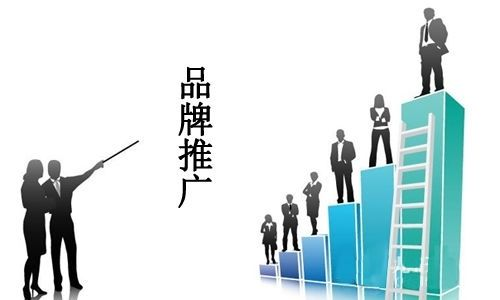免费咨询热线：023-6702-6306

想要品牌推广做得好，四项法则不能少！对于企业来说，在产品推广能够做好这四块，也是能够保证产品推广的效果，当然如果公司来说，做互联网产品推广不如选择一站式的互联网营销外包公司，利菲颖广告传媒专注互联网广告营销服务，旗下荣易传播广告平台，拥有优质的媒体资源，与国内知名企业（门户、自媒体、短视频平台等）合作，实现一站式广告投放，精准到达全网。

<<<<<<<<<<<<<<<<<<<<<<<<<<<<<<<<<<<<<<<<<<<<<<<<<<<<<<<<<<<<<<<<<<<<<<<<<<<<<<<<<<<<